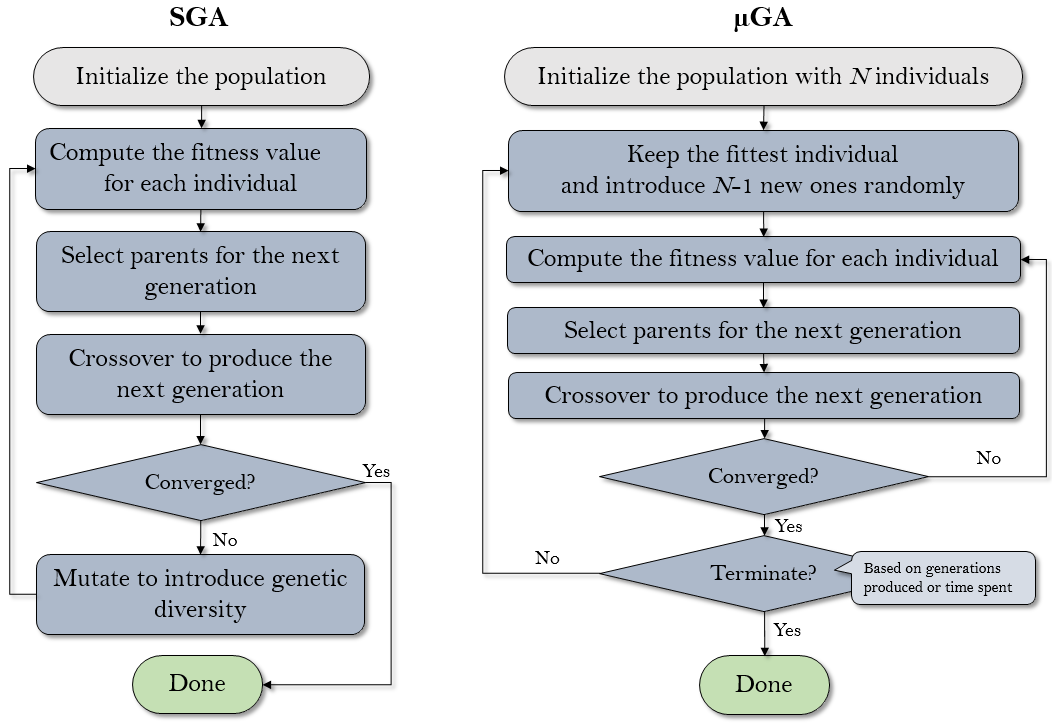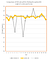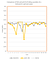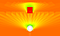# Micro Genetic Algorithm vs. Standard Genetic Algorithm

There are multiple ways to implement the genetic algorithm (GA). Earlier, I have implemented a standard GA (SGA) based on my understanding of the Haupts’ book. This week I have also implemented the micro genetic algorithm (µGA) that boasts potential higher performance as it needs only a small population at a time (hence “micro” in the name). Considering the fact that the calculation of the fitness value is the most compute-intensive part of GA in most applications in the real world (including those in Energy3D), a smaller population may result in less fitness calculation, hence allowing a better solution to be found within a given amount of time. The flowcharts in Figure 1 illustrate the main differences between SGA and µGA.Figure 1. The differences between SGA and µGA.

Does µGA work up to expectation? Let’s check it out using the two examples that I have written about earlier. The first example is concerned with finding the optimal tilt angle of a south-facing solar panel array on March 22 (a problem with a single maximum). I chose March 22 only for the purpose of demonstrating the algorithms with less calculations. The conclusion should not be affected if the annual output is chosen as the fitness function. Figure 2 shows the comparison of the results of twenty runs using SGA with a population of 20 after 5 generations, denoted by SGA(20, 5), with those using µGA with a population of 5 after 20 generations, denoted by µGA(5, 20). It seems µGA does have an advantage over SGA in this case as it produces more results that are close to the optimum.Figure 2. The comparison of the results of 20 runs using SGA with a population of 20 after 5 generations (denoted by SGA(20, 5)) with those using µGA with a population of 5 after 20 generations (denoted by µGA(5, 20)).

In the second example with regard to finding the optimal position of a single heliostat relative to the power tower, the results in Figure 3 seem to suggest that the performances of SGA(100, 5) and µGA(5, 100) are actually quite comparable. I chose a larger population for SGA and more generations for µGA because this application has a more complicated fitness landscape as shown in Figure 4.Figure 3. The comparison of the results of 20 runs using SGA with a population of 100 after 5 generations (denoted by SGA(100, 5)) with those using µGA with a population of 5 after 100 generations (denoted by µGA(5, 100)).

The cover image of this article shows a visualization of the fitness landscape in high resolution for the heliostat problem (high resolution means that the fitness function uses a fine-grained spatial grid and a small time step when computing the solar radiation on the field that the heliostat will be positioned). The results of Figure 3, however, are based on a much larger time step (in order to speed up the calculation for this investigation), as shown in Figure 4.Figure 4. A less accurate but faster numeric approximation for evaluating the fitness function was used in the calculation of the results shown in Figure 3. The discontinuities are the artifacts of using a time step of 30 minutes in solar radiation simulation, which may produce a lot of artificial local maxima.

In conclusion, both SGA and µGA can be used to solve optimization problems with acceptable performance for the examples discussed in this article. µGA may outperform SGA in some cases, but we don’t really know until we have tried both. Fortunately, you can use either µGA or SGA in Energy3D: µGA is automatically assumed when the user sets a population with less than nine individuals.

Computational Scientist, Physicist, & Inventor at the Institute for Future Intelligence https://intofuture.org